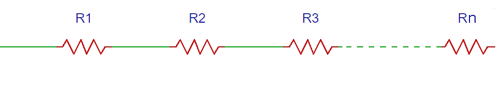# Resistors in Series CalculatorRTotal = R1 + R2 + R3 + ….. + Rn

 No of Resistors:

 Resistor 1 OhmKilo OhmMega Ohm Resistor 2 OhmKilo OhmMega Ohm

### Working of Resistors in Series Calculator

This series resistor calculator calculates the total resistance value for all the resistors connected in series. Just add the number of resistors in the first column, and then enter the values of each resistor with selecting the proper unit of resistor value in Ω, KΩ or MΩ.A series circuit is characterized as a circuit in which the same amount of current passes through all the resistors. As shown in the above diagram, n resistors are connected in series so the same current passes through every resistor and the total resistance must be equal to the sum of all the resistors in the series.

The formulae for the total resistance RTotal is as follows:

`RTotal = R1 + R2 + R3 + ….. + Rn`

Here you can find the calculator for resistors in parallel.